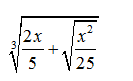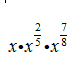#### MAT-HS.N-RN.02

 MAT-HS Targeted Standards(N) Concept: Number and Quantity(RN) Domain: The Real Number SystemCluster: Extend the properties of exponents to rational numbers MAT-HS.N-RN.02 Rewrite expressions involving radicals and rational exponents using the properties of exponents

## Student Learning Targets:

### Knowledge Targets

• I can understand that the denominator of a rational exponent is the root index and the numerator is the exponent of the radicand.

• I can
• I can

### Skills (Performance) Targets

• I can convert an expression in radical form to rational exponents and vice-versa.

• I can
• I can

## Alg II Re-write with Exponents Proficiency Scale

 Score Description Sample Activity 4.0 (advanced) In addition to Score 3.0, the student demonstrates in-depth inferences and applications regarding more complex material that go beyond end of instruction expectations. Simplify the following:3.5 In addition to Score 3.0 performance, the student demonstrates in-depth inferences and applications regarding the more complex content with partial success. 3.0 (proficient) The student can: apply properties of exponents to simplify expressions including rational exponents. Simplify the following expression.(41/3)3/4 (27p6)-5/3 2.5 The student demonstrates no major errors or omissions regarding the simpler details and processes (Score 2.0 content) and partial knowledge of the more complex ideas and processes (Score 3.0 content). 2.0 (progressing) There are no major errors or omissions regarding the simpler details and processes as the student can: perform operations with fractions. simplify fractions. recognize and recall specific terminology such as: exponents rational exponents However, the student exhibits major errors or omissions regarding the more complex ideas and processes. Simplify the following expressions. (p3)-2 (10/8)3 1.5 The student demonstrates partial knowledge of the simpler details and processes (Score 2.0 content) but exhibits major errors or omissions regarding the more complex ideas and procedures (Score 3.0 content). 1.0 (beginning) With help, the student demonstrates a partial understanding of some of the simpler details and processes (Score 2.0 content) and some of the more complex ideas and processes (Score 3.0 content). 0.5 With help, the student demonstrates a partial understanding of some of the simpler details and processes (Score 2.0 content) but not the more complex ideas and processes (Score 3.0 content). 0.0 Even with help, the student demonstrates no understanding or skill.